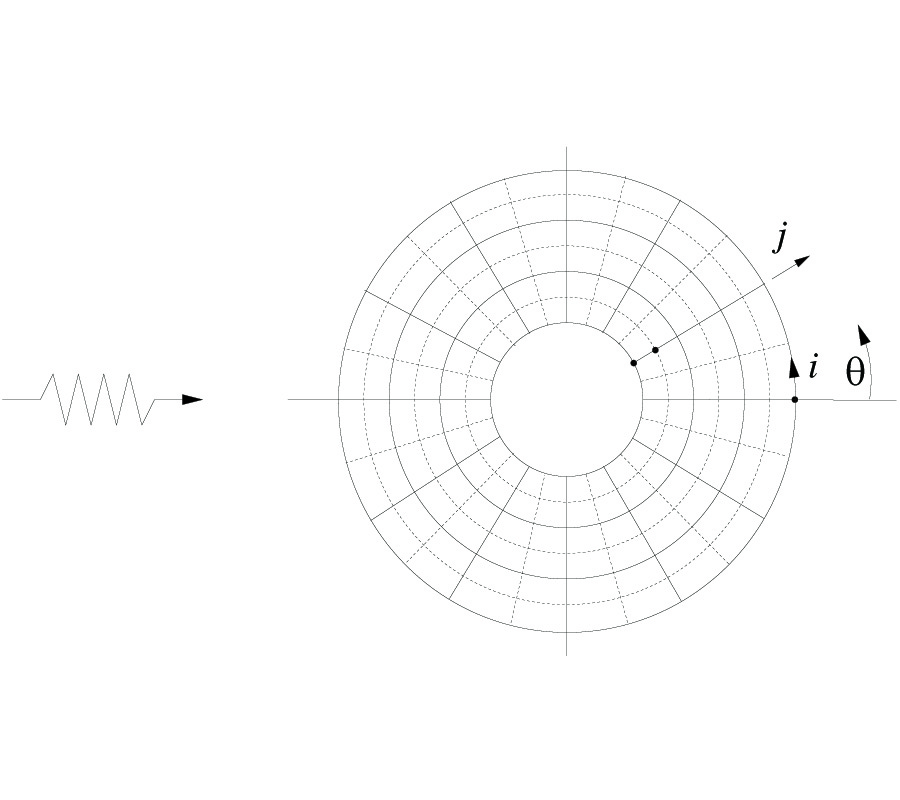# Vol. 23

Latest Volume
All Volumes
All Issues
2010-07-22

#### Cell-Vertex Based Multigrid Solution of the Time-Domain Maxwell's Equations

By Narendra Deore and Avijit Chatterjee
Progress In Electromagnetics Research B, Vol. 23, 181-197, 2010
doi:10.2528/PIERB10062002

## Abstract

The time domain Maxwell's equations are numerically solved using a multigrid method in a scattered field formulation and a cell-vertex based finite volume time domain framework. The multilevel method is an adaptation of Ni's  cell-vertex based multigrid technique, proposed for accelerating steady state convergence of nonlinear Euler equations of gas dynamics. Accelerated convergence to steady state of the time domain Maxwell's equations, for problems involving electromagnetic scattering, is obtained using multiple grids without the use of additional numerical damping usually required in nonlinear problems. The linear nature of the Maxwell's system also allows for a more accurate representation of the fine-grid problem on the coarse grid.

## Citation

Narendra Deore and Avijit Chatterjee, "Cell-Vertex Based Multigrid Solution of the Time-Domain Maxwell's Equations," Progress In Electromagnetics Research B, Vol. 23, 181-197, 2010.
doi:10.2528/PIERB10062002
http://test.jpier.org/PIERB/pier.php?paper=10062002

## References

1. Shankar, V., "A gigaflop performance algorithm for solving Maxwell's equations of electromagnetics," AIAA Paper, 584-590, 91--1578, June 1991.

2. Chatterjee, A. and R. S. Myong, "Efficient implementation of higher-order finite volume time-domain method for electrically large scatterers," Progress In Electromagnetics Research B, Vol. 17, 233-254, 2009.
doi:10.2528/PIERB09073102

3. Shang, J. S., "Characteristic-based algorithms for solving the Maxwell equations in the time domain," IEEE Antennas and Propagation Society Magazine, Vol. 37, No. 3, 15-25, 1995.
doi:10.1109/74.388807

4. Chatterjee, A. and A. Shrimal, "Essentially nonoscillatory finite volume scheme for electromagnetic scattering by thin dielectric coatings," AIAA Journal, Vol. 42, No. 2, 361-365, 2004.
doi:10.2514/1.553

5. Yee, K., "Numerical solutions of initial boundary value problems involving Maxwell's equations in isotropic media," IEEE Transactions on Antennas and Propagation, Vol. 14, 302-307, 1966.

6. Brandt, A., "Multi-level adaptive solutions to boundary-value problems," Mathematics of Computation, Vol. 31, No. 138, 333-390, 1977.
doi:10.1090/S0025-5718-1977-0431719-X

7. Turkel, E., "Progress in computational physics," Computers and Fluids, Vol. 11, No. 2, 121-144, 1983.
doi:10.1016/0045-7930(83)90006-3

8. Chatterjee, A. and G. Shevare, "A time-accurate multigrid algorithm for Euler equations," Fourteenth International Conference on Numerical Methods in Fluid Dynamics, Lecture Notes in Physics, 453, Springer-Verlag, 1995.

9. Ni, R.-H., "A multiple-grid scheme for solving the Euler equations," AIAA Journal, Vol. 20, 1565-1571, 1982.

10. Jameson, A., "Solution of the Euler equations for two-dimensional flow by a multigrid methods," Applied Mathematics and Computation, Vol. 13, 327-356, 1983.
doi:10.1016/0096-3003(83)90019-X

11. Deore, N. and A. Chatterjee, "A cell-vertex finite volume time domain method for electromagnetic scattering," Progress In Electromagnetics Research M, Vol. 12, 1-15, 2010.
doi:10.2528/PIERM10022003

12. Koeck, C., "Computation of three-dimensional flow using the Euler equations and a multiple-grid scheme," International Journal for Numerical Methods in Fluids, Vol. 5, 483-500, 1985.
doi:10.1002/fld.1650050507

13. Ni, R. H. and J. C. Bogoian, "Prediction of 3-D multistage turbine flow field using a multiple-grid Euler solver," AIAA Paper, 1-9, 89--0203, Jan. 1989.

14. Roe, P. L., "Fluctuations and signals --- A framework for numerical evolution problems," Numerical Methods in Fluid Dynamics, 219-257, K. W. Morton and M. J. Baines (eds.), Academic Press, 1982.

15. Hall, M. G., "Cell-vertex multigrid schemes for solution of the Euler equations," Numerical Methods for Fluid Dynamics II, 303-345, K. W. Morton and M. J. Baines (eds.), Clarendon Press, Oxford, 1985.

16. Radespiel, R., C. Rossow, and R. C. Swanson, "Efficient cell-vertex multigrid scheme for the three-dimensional Navier-Stokes equations," AIAA Journal, Vol. 28, No. 8, 1464-1472, 1990.
doi:10.2514/3.25239

17. Swanson, R. C. and R. Radespiel, "Cell centered and cell vertex multigrid schemes for the Navier-Stokes equations," AIAA Journal, Vol. 29, No. 5, 697-703, 1991.
doi:10.2514/3.10643

18. French, A. D., "Solution of the Euler equations on cartesian grids," Applied Numerical Mathematics, Vol. 49, 367-379, 2004.
doi:10.1016/j.apnum.2003.12.014

19. Shankar, V., W. F. Hall, and A. H. Mohammadin, "A time-domain differential solver for electromagnetic scattering problems," Proceedings of the IEEE, Vol. 77, No. 5, 709-721, 1989.
doi:10.1109/5.32061